## How to Calculate and Solve for Concentration Polarization | Corrosion

The image of concentration polarization is shown below.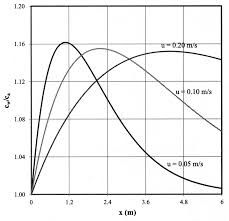To compute for concentration polarization, six essential parameters are needed and these parameters are Gas Constant (R), Temperature (T), Number of Electrons (n), Faraday’s Constant (F), Current Density (i) and Limiting Diffusion Current Density (iL).

The formula for calculating concentration polarization:

ηc = 2.3RT/nF log(1 – i/iL)

Where:

ηc = Concentration Polarization
R = Gas Constant
T = Temperature
n = Number of Electrons
i = Current Density
iL = Limiting Diffusion Current Density

Let’s solve an example;
Find the concentration Polarization when the gas constant is 14, the temperature is 7, the number of electrons is 8, the faraday’s constant is 4, the current density is 1 and the limiting diffusion current density is 2.

This implies that;

R = Gas Constant = 14
T = Temperature = 7
n = Number of Electrons = 8
F = Faraday’s Constant = 4
i = Current Density = 1
iL = Limiting Diffusion Current Density = 2

ηc = 2.3RT/nF log(1 – i/iL)
ηc = 2.3(14)(7)/(8)(4) log(1 – (1/2))
ηc = 225.39/32 log(1 – 0.5)
ηc = 7.043749 log(0.5)
ηc = 7.043749 (-0.301029)
ηc = -2.120

Therefore, the concentration polarization is -2.120.

## How to Calculate and Solve for Total Polarization | Electrical Properties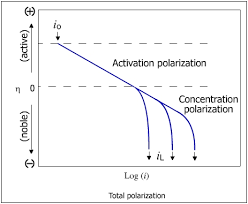The image of total polarization is shown above.

To compute for total polarization, three essential parameters are needed and these parameters are Electronic Polarization (Pe), Ionic Polarization (Pi) and Orientation Polarization (Po).

The formula for calculating total polarization:

P = Pe + Pi + Po

Where:

P = Total Polarization
Pe = Electronic Polarization
Pi = Ionic Polarization
Po = Orientation Polarization

Let’s solve an example;
Find the total polarization when the electronic polarization is 24, the ionic polarization is 4 and the orientation polarization is 2.

This implies that;

Pe = Electronic Polarization = 24
Pi = Ionic Polarization = 4
Po = Orientation Polarization = 2

P = Pe + Pi + Po
P = 24 + 4 + 2
P = 30

Therefore, the total polarization is 30 C/m².

Calculating the Electronic Polarization when the Total Polarization, the Ionic Polarization and the Orientation Polarization is Given.

Pe = P – Pi – Po

Where:

Pe = Electronic Polarization
P = Total Polarization
Pi = Ionic Polarization
Po = Orientation Polarization

Given an example;
Find the electronic polarization when the total polarization is 29, the ionic polarization is 10 and the orientation polarization is 3.

This implies that;

P = Total Polarization = 29
Pi = Ionic Polarization = 10
Po = Orientation Polarization = 3

Pe = P – Pi – Po
Pe = 29 – 10 – 3
Pe = 19 – 3
Pe = 16

Therefore, the electronic polarization is 16.

## How to Calculate and Solve for Polarization of Dielectric Medium | Electrical Properties

The image of polarization of dielectric medium is shown below.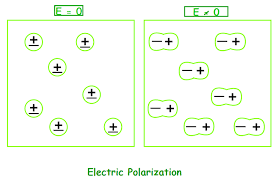To compute for polarization of dielectric medium, three essential parameters are needed and these parameters are Permittivity of Vacuum (εo), Dielectric Constant (εr) and Electric Field Intensity (E).

The formula for calculating polarization of dielectric medium:

P = εor – 1)E

Where:

P = Polarization of a Dielectric Medium
εo = Permittivity of Vacuum
εr = Dielectric Constant
E = Electric Field Intensity

Let’s solve an example;
Find the polarization of dielectric medium when the permittivity of vacuum is 12, the dielectric constant is 6 and the electric field intensity is 4.

This implies that;

εo = Permittivity of Vacuum = 12
εr = Dielectric Constant = 6
E = Electric Field Intensity = 4

P = εor – 1)E
P = (12)(6 – 1)(4)
P = (12)(5)(4)
P = 240

Therefore, the polarization of dielectric medium is 240 C/m².

## How to Calculate and Solve for Dielectric Displacement in Relation to Polarization | Electrical Properties

The image of dielectric displacement in relation to polarization is illustrated below.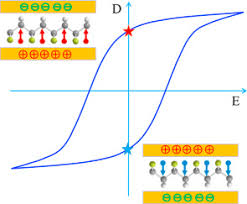To compute for dielectric displacement in relation to polarization, three essential parameters are needed and these parameters are Permittivity of Vacuum (εo), Electric Field Intensity (E) and Polarization (P).

The formula for calculating dielectric displacement in relation to polarization:

D = εoE + P

Where:

D = Dielectric Displacement in Relation to Polarization
εo = Permittivity of Vacuum
E = Electric Field Intensity
P = Polarization

Let’s solve an example;
Find the dielectric displacement in relation to polarization when the permittivity of vacuum is 8, the electric field intensity is 6 and the polarization is 2.

This implies that;

εo = Permittivity of Vacuum = 8
E = Electric Field Intensity = 6
P = Polarization = 2

D = εoE + P
D = (8)(6) + 2
D = (48) + 2
D = 50

Therefore, the dielectric displacement in relation to polarization is 50 C/m².

Calculating the Permittivity of Vacuum when the Dielectric Displacement in Relation to Polarization, the Electric Field Intensity and the Polarization is Given.

εo = D – P / E

Where:

εo = Permittivity of Vacuum
D = Dielectric Displacement in relation to polarization
E = Electric Field Intensity
P = Polarization

Let’s solve an example;
Find the permittivity of vacuum when the dielectric displacement in relation to polarization is 22, the electric field intensity is 6 and the polarization is 4.

This implies that;

D = Dielectric Displacement in relation to polarization = 22
E = Electric Field Intensity = 6
P = Polarization = 4

εo = D – P / E
εo = 22 – 4 / 6
εo = 18 / 6
εo = 3

Therefore, the permittivity of vacuum is 3.

## How to Calculate and Solve for Dielectric Displacement | Electrical Properties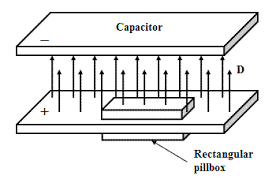The image of dielectric displacement is shown above.

To compute for dielectric displacement, two essential parameters are needed and these parameters are Permittivity of Vacuum (εo) and Electric Field Intensity (E).

The formula for calculating the dielectric displacement;

Do = εoE

Where:

Do = Dielectric Displacement
εo = Permittivity of Vacuum
E = Electric Field Intensity

Given an example;
Find the dielectric displacement when the permittivity of vacuum is 28 and the electric field intensity is 4.

This implies that;

εo = Permittivity of Vacuum = 28
E = Electric Field Intensity = 4

Do = εoE
Do = (28)(4)
Do = 112

Therefore, the dielectric displacement is 112 C/m².

Calculating the Permittivity of Vacuum when the Dielectric Displacement and the Electric Field Intensity is Given.

εo = DoE

Where:

εo = Permittivity of Vacuum
Do = Dielectric Displacement
E = Electric Field Intensity

Let’s solve an example;
Find the permittivity of vacuum when the dielectric displacement is 4 and the electric field intensity is 6.

This implies that;

Do = Dielectric Displacement = 4
E = Electric Field Intensity = 6

εo = DoE
εo = 4(6)
εo = 24

Therefore, the permittivity of vacuum is 24.

## How to Calculate and Solve for Electric Dipole Moment | Electrical Properties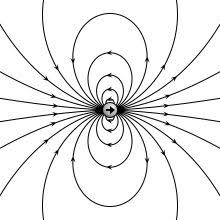The image of electric dipole moment is shown above.

To compute for electric dipole moment, two essential parameters are needed and these parameters are Magnitude of Each Dipole (q) and Distance of Separation (d).

The formula for calculating electric dipole moment:

p = qd

Where:

p = Electric Dipole Moment
q = Magnitude of Each Dipole
d = Distance of Separation

Let’s solve an example;
Find the electric dipole moment when the magnitude of each dipole is 21 and the distance of seperation is 7.

This implies that;

q = Magnitude of Each Dipole = 21
d = Distance of Separation = 7

p = qd
p = (21)(7)
p = 147

Therefore, the electric dipole moment is 147 C-m.

Calculating the Magnitude of Each Dipole when the Electric Dipole Moment and the Distance of Separation is Given.

q = p / d

Where:

q = Magnitude of Each Dipole
p = Electric Dipole Moment
d = Distance of Separation

Let’s solve an example;
Given that, the electric dipole moment is 12 and the distance of seperation is 4. Find the magnitude of each dipole?

This implies that;

p = Electric Dipole Moment = 12
d = Distance of Separation = 4

q = p / d
q = 12 / 4
q = 3

Therefore, the magnitude of each dipole is 3.

## How to Calculate and Solve for Dielectric Constant | Electrical Properties

The dielectric constant is illustrated by the image below.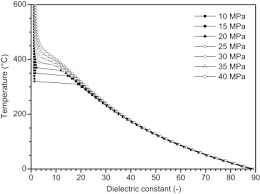To compute for dielectric constant, two essential parameters are needed and these parameters are Permittivity of Dielectric Material (ε) and Permittivity of Vacuum (εo).

The formula for calculating the dielectric constant:

εr = ε/εo

Where:

εr = Dielectric Constant
ε = Permittivity of Dielectric Material
εo = Permittivity of Vacuum

Given an example;
Find the dielectric constant when the permittivity of dielectric material is 36 and the permittivity of vacuum is 9.

This implies that;

ε = Permittivity of Dielectric Material = 36
εo = Permittivity of Vacuum = 9

εr = ε / εo
εr = 36 / 9
εr = 4

Therefore, the dielectric constant is 4.

Calculating the Permittivity of Dielectric Material when the Dielectric Constant and the Permittivity of Vacuum is Given.

ε = εro)

Where:

ε = Permittivity of Dielectric Material
εr = Dielectric Constant
εo = Permittivity of Vacuum

Let’s solve an example;
Find the permittivity of dielectric material when the dielectric constant is 14 and the permittivity of vacuum is 17.

This implies that;

εr = Dielectric Constant = 14
εo = Permittivity of Vacuum = 17

ε = εro)
ε = 14 (17)
ε = 238

Therefore, the permittivity of dielectric material is 238.

## How to Calculate and Solve for Capacitance of Parallel Plates | Electrical Properties

The image of capacitance of parallel plates is shown below.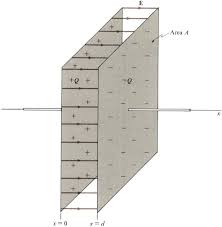To compute for capacitance of parallel plates, three essential parameters are needed and these parameters are Permittivity of the Material (ε), Cross-sectional (A) and Distance Between the Plates (d).

The formula for calculating capacitance of parallel plates:

C = εrεoA / d

Where:

C = Capacitance of Parallel Plates
εr = Permittivity of the material
εo = vacuum permittivity = 8.8541878176E-12
A = cross-sectional area
d = distance between the plates

Let’s solve an example;
Find the capacitance of parallel plates when the permittivity of the material is 14, the cross-sectional area is 8 and the distance between the plates is 2.

This implies that;

εr = Permittivity of the material = 14
εo = vacuum permittivity = 8.854E-12
A = cross-sectional area = 8
d = distance between the plates = 2

C = εrεoA / d
C = 14 x 8.854-12 x 8 / 2
C = 9.9166e-10 / 2
C = 4.958e-10

Therefore, the capacitance of parallel plates is 4.958e-10 farads.

## How to Calculate and Solve for Capacitance | Electrical Properties

The image of capacitance is shown below.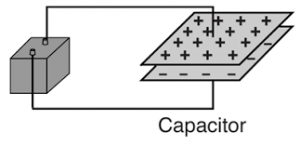To compute for capacitance, two essential parameters are needed and these parameters are Charge (Q) and Voltage (V).

The formula for calculating capacitance:

C = Q / V

Where;

C = Capacitance
Q = Charge
V = Voltage

Let’s solve an example;
Find the capacitance when the charge is 12 and the voltage is 6.

This implies that;

Q = Charge = 12
V = Voltage = 6

C = Q / V
C = 12 / 6
C = 2

Therefore, the capacitance is 2 farad.

Calculating the Charge when the Capacitance and the Voltage is Given.

Q = CV

Where;

Q = Charge
C = Capacitance
V = Voltage

Let’s solve an example;
Find the charge when the capacitance is 10 and the voltage is 5.

This implies that;

C = Capacitance = 10
V = Voltage = 5

Q = CV
Q = 10(5)
Q = 50

Therefore, the charge is 50.

## How to Calculate and Solve for Mobility of an Ionic Specie | Electrical Properties

The image of mobility of an ionic specie is shown below.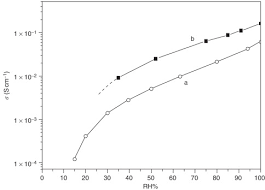To compute for mobility of an ionic specie, five essential parameters are needed and these parameters are Valence (nI), Electrical Charge (e), Diffusion Coefficient (DI), Boltzmann’s Constant (K) and Temperature (T).

The formula for calculating the mobility of an ionic specie:

μI = nIeDI/KT

Where:

μI = Mobility of an Ionic Specie
nI = Valence
e = Electrical Charge
DI = Diffusion Coefficient
K = Boltzmann’s Constant
T = Temperature

Let’s solve an example;
Find the mobility of an ionic specie when the valence is 18, the electrical charge is 9, the diffusion coefficient is 12, the boltzmann’s constant is 6 and the temperature is 3.

This implies that;

nI = Valence = 18
e = Electrical Charge = 9
DI = Diffusion Coefficient = 12
K = Boltzmann’s Constant = 6
T = Temperature = 3

μI = nIeDI/KT
μI = (18)(9)(12)/(6)(3)
μI = 1944/18
μI = 108

Therefore, the mobility of an ionic specie is 108 m²/(V. s).